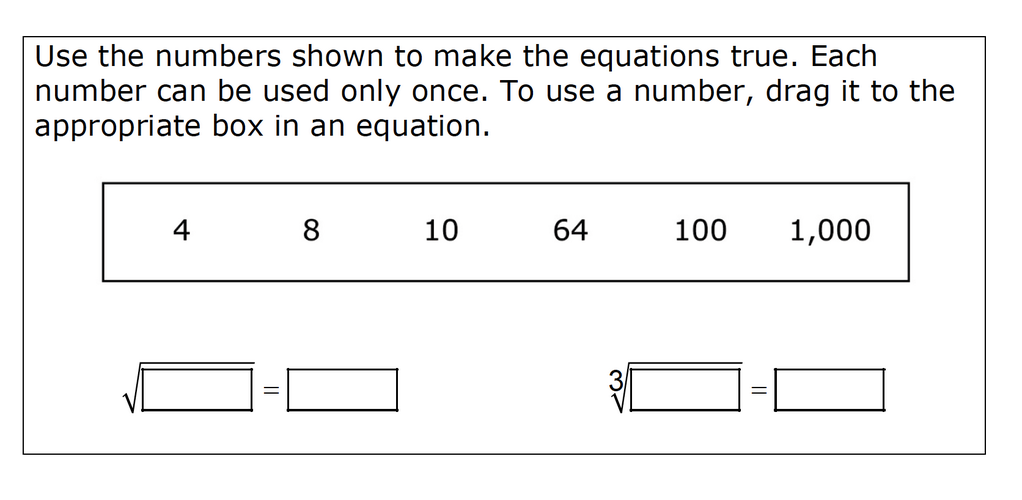Home > Grade 8 > Expressions & Equations > Solving Radical Equations

# Solving Radical Equations### Hint

Which numbers do you know the square/cube root of?  How does that help you? If you could use any number, what numbers would you use to make an equation?  How does that help you with the given numbers?

## Square Root Expression

Directions: Use the digits 1 to 9, at most one time each, to fill in …